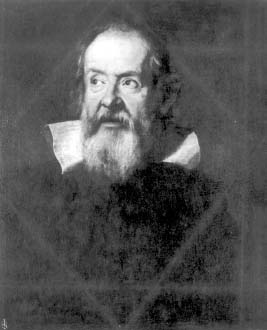NextPrevious

# What are permutations, combinations, and repeatables?

In order to perform certain probability problems, specific counting techniques need to be used, including determining the number of permutations, combinations, or repeatables. The following explains each term; the examples are based on a set of five cats on a shelf—a, b, c, d, and e, for convenience. (Note: The cats can be arranged in 120 ways, expressed as 5 × 4 × 3 × 2 × 1 = 5! [“5 factorial”] = 120).

The number of permutations is the number of different ways specific entities within the cat group can be arranged, with the positions being important. For example, given five cats, how many unique ways can they be placed in three positions on the shelf if position is important? The answers include ade, aed, dea, dae, ead, eda, abc, acb, bca, bac, etc.—a total of 60 ways. The notation for this is 5 P3 = 5!/(5 - 3)! = 5 × 4 × 3 × 2 × 1 / (2 × 1) = 5 × 4 × 3 = 60 (P in this case stands for permutations).Dutch mathematician and astronomer Christiaan Huygens devised the notion of the “gambler’s ruin.”Galileo Galilei, best known for his work as an astronomer, had already discovered the ideas of probability later restated as the “gambler’s ruin” by Christiaan Huygens.

Combinations mean the number of different ways specific entities can be grouped; but in this case, position does not matter. For example, in the problem of the cats, how many can be grouped into threes if position does not matter? The answers include abc, abd, abe, acd, ace, ade; but groupings such as cba are not allowed since it is equal to another combination: abc. The notation for this is 5 C3 = 5!/((5–3)! × 3!) = 5 × 4 × 3 × 2 × 1/(2 × 1 × 3 × 2 × 1) = 5 × 2 = 10 (C in this case stands for combinations).

With repeatables, position is important, too. But in this case, if one has five different cats, and many clones of each, how many unique ways can they be placed in three positions? This answer includes aaa, bbb, ccc, ddd, eee, eec, cee, etc.—a total of 125 ways. The notation for this is 5 R3 = 53 = 125 (R in this case stands for repeatables).

Close

This is a web preview of the "The Handy Math Answer Book" app. Many features only work on your mobile device. If you like what you see, we hope you will consider buying. Get the App